# JP4585048B2 - Endoscope system - Google Patents

## Info

Publication number
JP4585048B2
JP4585048B2 JP2010518448A JP2010518448A JP4585048B2 JP 4585048 B2 JP4585048 B2 JP 4585048B2 JP 2010518448 A JP2010518448 A JP 2010518448A JP 2010518448 A JP2010518448 A JP 2010518448A JP 4585048 B2 JP4585048 B2 JP 4585048B2
Authority
JP
Japan
Prior art keywords
unit
image
subject
probability
position
Prior art date
Legal status (The legal status is an assumption and is not a legal conclusion. Google has not performed a legal analysis and makes no representation as to the accuracy of the status listed.)
Active
Application number
JP2010518448A
Other languages
Japanese (ja)
Other versions
JPWO2010082383A1 (en
Inventor

Original Assignee
オリンパスメディカルシステムズ株式会社
Priority date (The priority date is an assumption and is not a legal conclusion. Google has not performed a legal analysis and makes no representation as to the accuracy of the date listed.)
Filing date
Publication date
Priority to JP2009007024 priority Critical
Priority to JP2009007024 priority
Application filed by オリンパスメディカルシステムズ株式会社 filed Critical オリンパスメディカルシステムズ株式会社
Priority to PCT/JP2009/067555 priority patent/WO2010082383A1/en
Application granted granted Critical
Publication of JP4585048B2 publication Critical patent/JP4585048B2/en
Publication of JPWO2010082383A1 publication Critical patent/JPWO2010082383A1/en
Application status is Active legal-status Critical
Anticipated expiration legal-status Critical

## Images

•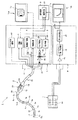•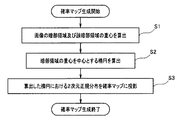•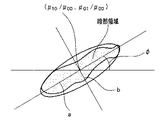•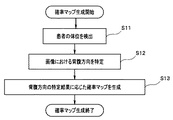•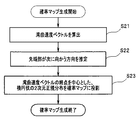•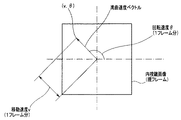•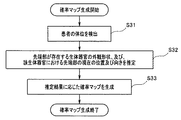•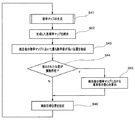•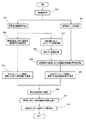•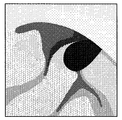•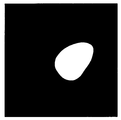•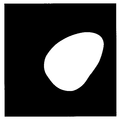•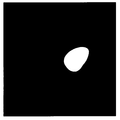••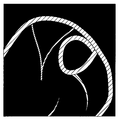•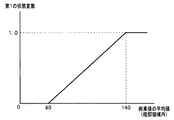•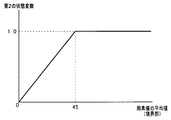•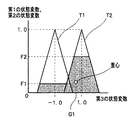•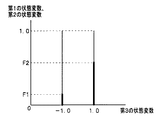## Classifications

• AHUMAN NECESSITIES
• A61MEDICAL OR VETERINARY SCIENCE; HYGIENE
• A61BDIAGNOSIS; SURGERY; IDENTIFICATION
• A61B1/00Instruments for performing medical examinations of the interior of cavities or tubes of the body by visual or photographical inspection, e.g. endoscopes; Illuminating arrangements therefor
• A61B1/04Instruments for performing medical examinations of the interior of cavities or tubes of the body by visual or photographical inspection, e.g. endoscopes; Illuminating arrangements therefor combined with photographic or television appliances
• AHUMAN NECESSITIES
• A61MEDICAL OR VETERINARY SCIENCE; HYGIENE
• A61BDIAGNOSIS; SURGERY; IDENTIFICATION
• A61B1/00Instruments for performing medical examinations of the interior of cavities or tubes of the body by visual or photographical inspection, e.g. endoscopes; Illuminating arrangements therefor
• A61B1/00147Holding or positioning arrangements
• AHUMAN NECESSITIES
• A61MEDICAL OR VETERINARY SCIENCE; HYGIENE
• A61BDIAGNOSIS; SURGERY; IDENTIFICATION
• A61B1/00Instruments for performing medical examinations of the interior of cavities or tubes of the body by visual or photographical inspection, e.g. endoscopes; Illuminating arrangements therefor
• A61B1/00147Holding or positioning arrangements
• A61B1/00158Holding or positioning arrangements using magnetic field

## Description

The present invention relates to an endoscope system, and more particularly to an endoscope system capable of supporting insertion of an endoscope in a subject such as a body cavity.

Endoscopes have been widely used in the medical field, industrial field, and the like. For example, in the medical field, endoscopes are used for performing observations and various treatments on living tissue in a body cavity.

In addition, a dark area in the observation image obtained by the endoscope is extracted, and the target position (when the endoscope is inserted so that the center position of the dark area becomes the center position of the observation image) A technique for appropriately setting the movement direction and the movement amount) is disclosed in Japanese Patent Laid-Open No. 7-155289.

In general, when performing the insertion operation of the endoscope, the surgeon or the like directly from the observation image in addition to various information that can be directly read from the observation image obtained by the endoscope. As a result of considering various other elements that cannot be read and integrated, a target position for directing the distal end portion of the endoscope is appropriately determined. Specifically, the operator, for example, confirms the situation around the distal end portion of the endoscope while viewing the observation image obtained by the endoscope, and further determines the bending speed and coverage of the endoscope. By considering each element such as the examiner's body in an integrated manner, it is determined as appropriate where the tip portion should be directed next.

On the other hand, the technique disclosed in Japanese Patent Application Laid-Open No. 7-155289 is obtained by the endoscope when setting the target position of the distal end portion of the endoscope disposed in the body cavity. It can be said that the calculation is merely performed depending on the extraction result of the dark area in the observation image, that is, the calculation is performed while substantially ignoring various elements other than the extraction result of the dark area.

As a result, in the technique disclosed in Japanese Patent Application Laid-Open No. 7-155289, the accuracy of the result of setting the target position for directing the distal end portion of the endoscope is lowered, so that the endoscope There is a problem that it cannot be inserted smoothly.

The present invention has been made in view of the above-described circumstances, and an object of the present invention is to provide an endoscope system that can smoothly insert an endoscope as compared with the conventional art.

The endoscope system according to the present invention includes an imaging unit that images a subject, a position detection unit that detects a position indicating a predetermined target in the image of the subject obtained by the imaging unit, and the image captured by the imaging unit. A probability value as a degree indicating the accuracy of the position being the predetermined target using the first information obtained from the image and the second information regarding the state of the subject imaged by the imaging unit And a probability calculating unit for calculating.

An endoscope system according to the present invention includes an insertion portion that is inserted into a body cavity, an endoscope that includes an imaging portion provided at a distal end portion of the insertion portion, and a target position through which the distal end portion passes. A position setting unit set in the image of the subject obtained by the imaging unit, first information obtained from the image captured by the imaging unit, and second information relating to the state of the subject captured by the imaging unit And a probability calculation unit that calculates a probability value as a degree indicating the accuracy of the position being the predetermined target.

An endoscope system according to the present invention includes an imaging unit that images a subject, a position detection unit that detects a position indicating a predetermined target in the image of the subject obtained by the imaging unit, and a plurality of pixel features from the image A pixel feature amount calculation unit that acquires an amount; and a probability calculation unit that calculates a probability value as a degree indicating the accuracy of the position being the predetermined target based on the plurality of pixel feature amounts; It is characterized by having.

An endoscope system according to the present invention includes an imaging unit that moves inside a subject and acquires an image of a subject inside the subject, and an extraction unit that extracts a dark region in an image corresponding to the subject image. The information acquisition unit for acquiring predetermined information different from the extraction result of the dark area by the extraction unit, and the probability value indicating the accuracy as the passing target position of the imaging unit, the extraction result of the dark area by the extraction unit And a probability calculating unit that calculates using the predetermined information.

An endoscope system according to the present invention includes an insertion unit that is inserted into a body cavity of a subject, and an imaging unit that is provided at a distal end portion of the insertion unit and acquires an image of a subject in the body cavity. An endoscope, an extraction unit that extracts a dark area in an image corresponding to the image of the subject, an information acquisition unit that acquires predetermined information different from the extraction result of the dark area by the extraction unit, and a passage through the imaging unit And a probability calculation unit that calculates a probability value indicating accuracy as a target position using the extraction result of the dark region by the extraction unit and the predetermined information.

An endoscope system according to the present invention includes an imaging unit that moves inside a subject and obtains an image of a subject inside the subject, and an image corresponding to a passing target position of the imaging unit according to the image of the subject. A position setting unit that is set as one pixel position in the dark region, a state variable calculation unit that calculates a state variable indicating the authenticity of the dark region, and an accurate determination of the passing target position based on the state variable And a probability calculation unit for calculating a probability value indicating the length.

An endoscope system according to the present invention includes an insertion unit that is inserted into a body cavity of a subject, and an imaging unit that is provided at a distal end portion of the insertion unit and acquires an image of a subject in the body cavity. A endoscope, a position setting unit that sets a target position of passage of the tip portion as one pixel position in a dark region of an image corresponding to the image of the subject, and a state variable that indicates a degree of authenticity of the dark region And a probability calculation unit for calculating a probability value indicating the accuracy of the passing target position based on the state variable.

The figure which shows an example of a structure of the principal part of the endoscope system which concerns on embodiment of this invention. The figure which shows an example of the process performed when producing | generating a 1st probability map. The figure which shows an example of the ellipse which represents a dark part area | region approximately. The figure which shows an example of the process performed when producing | generating a 2nd probability map. The figure which shows an example of the process performed when producing | generating a 3rd probability map. The figure which showed the correlation of moving speed v, rotational speed (theta), and a bending speed vector. The figure which shows an example of the process performed when producing | generating a 4th probability map. The figure which shows an example of the process performed when setting a curvature target position using a probability map. The figure which shows an example of the process performed when calculating the reliability of the dark part area | region which exists in an original image. The figure which shows an example of the original image used for the process of FIG. The figure which shows an example of the dark part area | region mask image produced | generated using the original image of FIG. The figure which shows an example of the expansion | swelling image obtained by applying a morphological process with respect to the dark part area | region mask image of FIG. The figure which shows an example of the shrinkage | contraction image obtained by applying a morphological process with respect to the dark part area | region mask image of FIG. The figure which shows an example of the boundary mask image produced | generated using the expansion image of FIG. 12, and the shrinkage | contraction image of FIG. The figure which shows an example of the edge image produced | generated using the original image of FIG. The figure which shows an example of the 1st membership function used when acquiring the 1st state variable F1. The figure which shows an example of the 2nd membership function used when acquiring the 2nd state variable F2. The schematic diagram which showed a mode that the 3rd state variable G1 was calculated by applying the 1st state variable F1 and the 2nd state variable F2 to an output fuzzy set. The figure which shows the example of the output fuzzy set different from FIG.

Hereinafter, embodiments of the present invention will be described with reference to the drawings.

1 to 19 relate to an embodiment of the present invention. FIG. 1 is a diagram illustrating an example of a configuration of a main part of an endoscope system according to an embodiment of the present invention. FIG. 2 is a diagram illustrating an example of processing performed when generating the first probability map. FIG. 3 is a diagram illustrating an example of an ellipse that approximately represents a dark area. FIG. 4 is a diagram illustrating an example of processing performed when the second probability map is generated. FIG. 5 is a diagram illustrating an example of processing performed when the third probability map is generated. FIG. 6 is a diagram showing the correlation among the moving speed v, the rotational speed θ, and the bending speed vector. FIG. 7 is a diagram illustrating an example of processing performed when the fourth probability map is generated. FIG. 8 is a diagram illustrating an example of processing performed when the bending target position is set using the probability map.

FIG. 9 is a diagram illustrating an example of processing performed when the reliability of the dark area existing in the original image is calculated. FIG. 10 is a diagram illustrating an example of an original image used in the process of FIG. FIG. 11 is a diagram illustrating an example of a dark area mask image generated using the original image of FIG. 12 is a diagram illustrating an example of an expanded image obtained by applying a morphological process to the dark area mask image of FIG. FIG. 13 is a diagram illustrating an example of a contracted image obtained by applying morphological processing to the dark area mask image of FIG. 11. FIG. 14 is a diagram illustrating an example of a boundary mask image generated using the dilated image of FIG. 12 and the contracted image of FIG. FIG. 15 is a diagram illustrating an example of an edge image generated using the original image of FIG. FIG. 16 is a diagram illustrating an example of a first membership function used when acquiring the first state variable F1. FIG. 17 is a diagram illustrating an example of the second membership function used when acquiring the second state variable F2. FIG. 18 is a schematic diagram showing how the third state variable G1 is calculated by applying the first state variable F1 and the second state variable F2 to the output fuzzy set. FIG. 19 is a diagram illustrating an example of an output fuzzy set different from that in FIG.

As shown in FIG. 1, the endoscope system 1 is inserted into a body cavity of a patient as a subject, and is provided in an endoscope 2 that captures an image of a subject in the body cavity, and the endoscope 2. The attached and removable connector 14, the sense coil unit 7 disposed in the periphery of the bed on which the patient lies, the endoscope insertion shape detection device 8, the terminal device 9, and the monitors 10 a and 10 b. And is configured.

In addition, the processor 6 performs signal processing on the light source unit 3 that supplies illumination light for illuminating the subject to be imaged to the endoscope 2 and the imaging signal output from the endoscope 2. A signal processing unit 4 that generates and outputs a video signal, a bending control unit 5 that performs bending control on the endoscope 2, and a source coil driving unit 43.

The endoscope 2 includes an elongated insertion portion 11 to be inserted into a body cavity of a subject, an operation portion 12 provided at the rear end of the insertion portion 11, and a universal cord 13 extended from the operation portion 12. Have. A connector 14 that can be attached to and detached from the processor 6 is provided at the rear end of the universal cord 13.

The insertion unit 11 moves in the body cavity of the subject according to an insertion operation by an operator or the like. The insertion portion 11 includes a hard distal end portion 15 provided on the distal end side, a bending portion 16 connected to the rear end of the distal end portion 15, and between the rear end of the bending portion 16 and the front end of the operation portion 12. And a flexible tube portion 17 having flexibility. In addition, n source coils C1, C2,... Cn that generate a magnetic field corresponding to the source coil drive signal applied by the source coil drive unit 43 are provided at substantially equal intervals in the insertion unit 11. ing.

The distal end portion 15 is provided with an imaging unit 15a including an objective optical system that forms an image of the subject, and an imaging element that outputs the image of the subject formed through the objective optical system as an imaging signal. It has been.

The operation unit 12 includes a scope switch 18 that gives an instruction to acquire a freeze image (still image) and the like, and a bending mode changeover switch 19 that gives an instruction to switch the bending mode of the bending unit 16 to either the manual mode or the automatic mode. And a bending joystick 20 for instructing a bending direction and a bending angle of the bending portion 16 when the manual mode is selected. In addition, a treatment instrument insertion port 39 that leads to a treatment instrument channel (not shown) through which a treatment instrument or the like can be inserted is provided on the rear end side of the flexible tube section 17 and in the vicinity of the front end of the operation section 12. Yes.

A light guide 21 that transmits illumination light supplied from the light source unit 3 to the distal end portion 15 is inserted into the insertion portion 11 and the like of the endoscope 2.

One end face (incident end face) of the light guide 21 is disposed so as to protrude from the connector 14. Further, the other end face (outgoing end face) of the light guide 21 is disposed in the vicinity of an illumination optical system (not shown) provided at the tip portion 15. With such a configuration, the illumination light supplied from the light source unit 3 becomes an imaging target of the imaging unit 15a after passing through the light guide 21 and an illumination optical system (not shown) in a state where the connector 14 is connected to the processor 6. Illuminate the subject.

The light source unit 3 having a function as a light source device includes, for example, a lamp 22 that emits illumination light that is white light, a lamp driving unit 23 that supplies power required to drive the lamp 22, a diaphragm 24, and signal processing. A diaphragm control unit 25 that increases or decreases the diaphragm amount (aperture amount) of the diaphragm 24 based on the video signal output from the unit 4, and supplies the illumination light that has passed through the diaphragm 24 to the incident end face of the light guide 21. And a condensing optical system 26.

The aperture control unit 25 is a value obtained by, for example, calculating the average brightness based on the luminance component of the input video signal and then subtracting a reference value corresponding to an appropriate brightness from the average brightness. The amount of illumination light passing through the diaphragm 24 is appropriately changed by increasing or decreasing the diaphragm amount (aperture amount) of the diaphragm 24 based on the difference value.

The signal processing unit 4 performs signal processing on the image sensor driving unit 36 that outputs an image sensor driving signal for driving the image sensor provided in the image capturing unit 15a, and the image signal output from the image capturing unit 15a. Thus, a video processing unit 37 that generates and outputs a video signal is provided. As a result, an endoscopic image Ia corresponding to the video signal is displayed on the monitor 10a.

When the bending mode of the bending portion 16 is switched to the manual mode based on an instruction given by the bending mode changeover switch 19, the bending control unit 5 changes the bending portion based on the inclination direction and the inclination amount of the bending joystick 20. Control for changing 16 bending directions and bending angles is performed. Further, the bending control unit 5 having a function as a drive control unit, when the bending mode of the bending unit 16 is switched to the automatic mode based on an instruction given by the bending mode changeover switch 19, Based on the calculation result, control for changing the bending direction and the bending angle of the bending portion 16 is performed.

The source coil driving unit 43 is connected to n source coils C1, C2,... Cn provided in the insertion unit 11, and sequentially supplies an AC source coil driving signal to each of the source coils. Apply. As a result, an alternating magnetic field is generated around each source coil provided in the insertion portion 11.

The sense coil unit 7 is provided with a sense coil group 44 that detects magnetic fields emitted from the n source coils C1, C2,... Cn provided inside the insertion portion 11 and outputs them as magnetic field detection signals. It has been.

The endoscope insertion shape detection device 8 includes an amplifier 45 that amplifies the magnetic field detection signal output from the sense coil unit 7, and n source coils C1, C2,... Based on the magnetic field detection signal output from the amplifier 45. ..Source coil position / orientation detection unit 46 that detects the three-dimensional coordinate position and orientation of Cn and outputs them as insertion shape information, and an insertion unit based on the insertion shape information output from the source coil position / orientation detection unit 46 An insertion shape estimation unit 47 that estimates 11 insertion shapes and outputs them as insertion shape image signals. Thereby, the insertion shape image Ib of the insertion part 11 according to the said insertion shape image signal is displayed on the monitor 10b.

The terminal device 9 is performed when the bending mode of the bending unit 16 is the automatic mode based on the video signal output from the video processing unit 37, the insertion shape image signal output from the insertion shape estimation unit 47, and the like. An arithmetic processing unit 91 that performs calculation related to bending control and outputs the calculation result to the bending control unit 5 is provided. The specific contents of the calculation performed in the calculation processing unit 91 will be described later.

In addition, the terminal device 9 is provided with a memory (not shown) that can temporarily store the calculation results in the calculation processing unit 91.

Next, the operation of the endoscope system 1 will be described. In the following, description of control when the bending mode changeover switch 19 is switched to the manual bending mode is omitted, and control when the bending mode changeover switch 19 is switched to the automatic bending mode is mainly described. Shall be performed.

First, after connecting and starting each part of the endoscope system 1, the surgeon inserts the insertion part 11 of the endoscope 2 into the body cavity of the patient and switches the bending mode switch 19 to the automatic bending mode. In response to this, imaging of the subject in the imaging unit 15a of the endoscope 2 is started, and a magnetic field is started to be generated in each source coil provided in the insertion unit 11.

An imaging signal output from the imaging unit 15a when the subject is imaged is output to the processor 6 via the universal code 13 and the connector 14, and after being converted into a video signal by the video processing unit 37, the arithmetic processing of the terminal device 9 is performed. Input to the unit 91. In addition, the magnetic field detection signal output from the sense coil unit 7 with the generation of the magnetic field in each source coil provided in the insertion unit 11 is amplified by the amplifier 45, and each source is detected by the source coil position / orientation detection unit 46. It is converted as the three-dimensional coordinate information of the coil, and after the insertion shape estimation unit 47 estimates the insertion shape, it is input to the arithmetic processing unit 91 of the terminal device 9 as an insertion shape image signal.

The arithmetic processing unit 91 of the terminal device 9 sets and sets a bending target position (passing target position) that is a passing target of the distal end portion 15 by performing processing based on an input video signal, an insertion shape image signal, and the like. Information on the target bending position is output to the bending control unit 5.

Here, a process performed by the arithmetic processing unit 91 when setting the above-described bending target position will be described.

The arithmetic processing unit 91 having a function as an extraction unit calculates a dark part region of an image (endoscopic image) corresponding to an input video signal and a center of gravity of the dark part region (step S1 in FIG. 2). .

The center of gravity of the dark area calculated in step S1 in FIG. 2 coincides with the center of the moment of the pixel value of the dark area, that is, the following formula (1) is used as a method for calculating the moment of the digital image. Thus, the pixel position is calculated as (μ10 / μ00, μ01 / μ00).

After that, the arithmetic processing unit 91 calculates the dark part region calculated in step S1 of FIG. 2 based on the second moments μ20, μ11, and μ02 of the pixel value of the dark part region and the zero order moment μ00 of the pixel value of the dark part region. An ellipse centered on the center of gravity (μ10 / μ00, μ01 / μ00) is calculated (step S2 in FIG. 2).

Specifically, the arithmetic processing unit 91 has, for example, a shape as shown in FIG. 3 based on the second moments μ20, μ11, and μ02 of the pixel values in the dark area and the 0th moment μ00 of the pixel values in the dark area. The calculation result of the dark area is provided with center coordinates (μ10 / μ00, μ01 / μ00), a major axis length a, a minor axis length b, and a major axis inclination φ with respect to the horizontal direction of the image. Then, an ellipse that approximately represents the dark area is calculated. The major axis length a, the minor axis length b, and the major axis inclination φ with respect to the horizontal direction of the image are respectively expressed by the following mathematical formulas (2) to (4).

Then, the arithmetic processing unit 91 projects the two-dimensional normal distribution in the ellipse calculated in step S2 of FIG. 2 on the probability map (step S3 of FIG. 2).

That is, the arithmetic processing unit 91 generates the first probability map corresponding to the pixel value of the endoscopic image obtained by the endoscope 2 by performing the processing of step S1 to step S3 in FIG.

Note that each probability map generated in the present embodiment is obtained by assigning one probability value to one pixel of the endoscopic image.

In addition, the first probability map described above is not limited to the one generated by the processing of Steps S1 to S3 in FIG. 2. For example, the probability value of the bright area is relatively low, and the dark area is It may be generated by applying a predetermined function that makes the probability value relatively high to each pixel value of the endoscopic image. Specifically, for example, when the probability value corresponding to the pixel value C (x, y) of the original image is P (x, y), the first probability map described above uses the following formula (5). May be generated.

It should be noted that the pixel value C (x, y) in the above equation (5) takes a value between 0 and 255.

On the other hand, the arithmetic processing unit 91 having a function as an information acquisition unit detects the position of the patient at present based on a predetermined input signal including information on at least the patient's dorsoventral direction (FIG. 4 step S11).

The predetermined input signal may be a signal output in accordance with a detection result of a position and orientation of a coil (not shown) attached to the patient, or a position selection switch provided in the scope switch 18 or the like It may be a signal that is output in accordance with the content of the instruction.

Thereafter, the arithmetic processing unit 91, based on the detection result in step S11 of FIG. 4 and the insertion shape image signal, the dorsoventral direction (dorsal and abdominal) in the image (endoscopic image) corresponding to the input video signal Side) is specified (step S12 in FIG. 4).

By the way, when a general endoscope insertion operation is considered, it can be assumed that it is relatively rare to curve the distal end portion of the endoscope toward the abdomen or back of the patient. The arithmetic processing unit 91 corresponds to the dorsal side and the ventral side of the endoscopic image based on the first algorithm constructed in accordance with such an assumption and the specific result in step S12 of FIG. A probability map in which the probability of the region is set relatively low is generated (step S13 in FIG. 4).

That is, the arithmetic processing unit 91 generates a second probability map corresponding to the current posture of the patient into which the endoscope 2 is inserted by performing the processes of steps S11 to S13 in FIG.

On the other hand, the arithmetic processing unit 91 having a function as an information acquisition unit calculates a bending speed vector corresponding to the moving speed of the distal end portion 15 (imaging unit 15a), for example, by reading the control content of the bending control unit 5 ( Step S21 in FIG.

Specifically, based on the control content of the bending control unit 5, the arithmetic processing unit 91 starts the center of the endoscopic image on the image plane of the endoscopic image, and the distal end portion 15 is within one frame. A bending speed vector where v is a moving speed indicating the amount of movement moved to, and θ is a rotating speed indicating the amount of rotation of the distal end portion 15 during one frame on the image plane of the endoscopic image. calculate. Note that the moving speed v, the rotational speed θ, and the bending speed vector described above are, for example, as shown in FIG. 6 in the endoscopic image of the current frame.

Thereafter, the arithmetic processing unit 91 sets the direction in which the distal end portion 15 is directed to the next direction based on the calculation result of the bending velocity vector obtained in step S21 of FIG. 5 (the center of the endoscopic image). Is estimated as a direction from to the end point (step S22 in FIG. 5).

By the way, when considering a bending operation of a general endoscope, an image position when the bending portion is actually driven to bend and a speed, that is, an end position of the above-described bending speed vector, a bending target position. Can be assumed to exist.

Then, based on the second algorithm constructed in accordance with such an assumption and the estimation result in step S22 of FIG. 5, the arithmetic processing unit 91 ends the bending velocity vector in the endoscopic image of the current frame. An elliptical two-dimensional normal distribution centered on (v, θ) is projected onto the probability map (step S23 in FIG. 5).

That is, the arithmetic processing unit 91 generates the third probability map corresponding to the bending speed of the endoscope 2 inserted into the body cavity of the patient by performing the processing of step S21 to step S23 of FIG. .

On the other hand, the arithmetic processing unit 91 detects the position of the patient at present by performing the same process as the process described as step S11 in FIG. 4 (step S31 in FIG. 7).

Thereafter, the arithmetic processing unit 91, based on the detection result in step S31 of FIG. 7 and the insertion shape image signal, the external shape of the living organ in which the distal end portion 15 exists, and the current state of the distal end portion 15 in the living organ. The position and orientation are estimated (step S32 in FIG. 7).

And the arithmetic processing part 91 produces | generates the probability map according to the estimation result in FIG.7 S32 (FIG.7 S33). Specifically, for example, when the arithmetic processing unit 91 obtains an estimation result that the current position of the distal end portion 15 is the descending colon and the orientation of the distal end portion 15 faces the patient's head, A probability map is generated in which the probability of the area corresponding to the front and substantially the front of the unit 15 is set relatively high.

In other words, the arithmetic processing unit 91 generates the fourth probability map corresponding to the current position and orientation of the distal end portion 15 by performing the processing in steps S31 to S33 in FIG.

On the other hand, the arithmetic processing unit 91 projects a two-dimensional normal distribution centered on the one curve target position based on the one curve target position set in the past (for example, the curve target position set last time). 5 probability maps are generated.

And the arithmetic processing part 91 performs the process which produces | generates the 1st probability map-the 5th probability map mentioned above as a process shown to step S41 of FIG.

Next, the arithmetic processor 91 performs a process for integrating the probability maps generated in the process of step S41 of FIG. 8 (step S42 of FIG. 8).

Specifically, the arithmetic processing unit 91 sets P1 as the probability value of one position (pixel position) in the first probability map, P2 as the probability value of the one position in the second probability map, and the third probability. When the probability value of the one position in the map is P3, the probability value of the one position in the fourth probability map is P4, and the probability value of the one position in the fifth probability map is P5, The integrated value Cx at one position is calculated by the following mathematical formula (6).

In the above equation (6), μa represents a weighting value for the first probability map, μb represents a weighting value for the second probability map, μc represents a weighting value for the third probability map, and μd is A weight value for the fourth probability map is indicated, and μe indicates a weight value for the fifth probability map.

Further, the integrated value Cx is not limited to the one calculated by the weighting calculation as shown in the above formula (6), and is calculated by using another calculation method such as a fuzzy logic calculation or a mixed expert model, for example. It may be a thing.

Thereafter, the arithmetic processing unit 91 performs the process of calculating the integrated value Cx using the above formula (6) for all positions (pixel positions) in one screen, and then the calculated integrated value Cx is 0. Normalization processing is performed so that the value is 1 or less.

Then, by performing the process as described above as the process of step S42 in FIG. 8, a new probability obtained by integrating the five probability maps including the first probability map to the fifth probability map. A map is generated.

In other words, the integrated value Cx after the above-described normalization processing is performed corresponds to a probability value indicating the accuracy as the bending target position (the passing target position of the tip 15 or the imaging unit 15a).

The arithmetic processing unit 91 extracts the position (pixel position) having the highest probability value from the integrated probability map generated by the process of step S42 in FIG. 8 (step S43 in FIG. 8), and then extracts the position (pixel position). ) Is present (step S44 in FIG. 8).

When there are a plurality of positions extracted by the process of step S43 in FIG. 8, the arithmetic processing unit 91 calculates the centroid (center of moment) of the probability values in the probability map after integration (step S45 in FIG. 8), A position (pixel position) corresponding to the center of gravity is set as a bending target position (step S46 in FIG. 8).

In addition, when there is only one position extracted by the process of step S43 in FIG. 8, the arithmetic processing unit 91 sets the position (pixel position) as a bending target position (step S46 in FIG. 8).

Then, the arithmetic processing unit 91 outputs the information on the bending target position set in the processing of step S46 in FIG. 8 to the bending control unit 5, and then repeats a series of processing from step S41 in FIG. 8 again.

As described above, the endoscope system 1 according to the present embodiment accurately performs the series of processes related to FIGS. 2 to 8 in the automatic bending mode, thereby accurately setting the target position toward the distal end portion of the endoscope. Can be calculated. As a result, the endoscope system 1 of the present embodiment can make the insertion of the endoscope smoother than before.

In addition, according to a series of processes shown in FIGS. 2-8, it is not restricted to creating the integrated probability map using all of the first probability map to the fifth probability map. An integrated probability map may be created using only the probability map and the second probability map.

In addition, the fifth probability map generated in the series of processes shown in FIGS. 2 to 8 is not limited to the one generated based on the one curve target position set in the past, for example, created last time. The integrated probability map may be used as it is.

On the other hand, according to the endoscope system 1 of the present embodiment, a series of processes relating to FIGS. 9 to 19 may be performed in the automatic bending mode in order to smoothly insert the endoscope.

First, the arithmetic processing unit 91 acquires, for example, an original image as shown in FIG. 10 corresponding to the input video signal (step S51 in FIG. 9).

Thereafter, the arithmetic processing unit 91 extracts a dark part region existing in the original image obtained in step S51 of FIG. 9 (step S52 of FIG. 9). Specifically, for example, the arithmetic processing unit 91 extracts an isolated region having a pixel value less than a predetermined value from the original image obtained in step S51 of FIG. 9 as a dark region. Then, by performing such processing, the arithmetic processing unit 91 uniformly sets the pixel values of the dark area to 255 and sets the pixel values of the non-dark area to 0 uniformly from the original image shown in FIG. Then, a dark area mask image as shown in FIG. 11 is generated.

The arithmetic processing unit 91 considers each pixel existing in the area masked by the dark area mask image shown in FIG. 11 in the original image shown in FIG. 10 as a pixel in the dark area, An average value of the pixel values of all the pixels is calculated (step S53 in FIG. 9).

Thereafter, the arithmetic processing unit 91 applies the average value of the pixel values of all the pixels existing in the dark area calculated by the process of step S53 of FIG. 9 to the first membership function shown in FIG. The first state variable F1 used for the fuzzy logic calculation is acquired (step S54 in FIG. 9).

The first membership function described above is a function in which the darkness level of the dark area extracted by the processing of steps S52 and S53 in FIG. 9 is represented by a value of 0 ≦ F1 ≦ 1, and the dark area is It is set to take a value close to F1 = 0 when darker, and to take a value close to F1 = 1 when the dark area is brighter. Specifically, as shown in FIG. 16, the first membership function in the present embodiment is F1 = 0 when the average value of all the pixel values in the dark area is 40 or less, and 140 In the above case, F1 = 1 is set, and when it exceeds 40 and is less than 140, it is set to increase linearly in 0 <F1 <1.

Further, the arithmetic processing unit 91 applies the morphological process to the dark area mask image generated in step S52 of FIG. 9 (step S55 of FIG. 9). Then, the arithmetic processing unit 91 performs the process of step S55 of FIG. 9 to expand the dark part area (area of pixel value = 255) extracted in step S52 of FIG. 9 and the dark part area. A contracted contracted image is acquired. Note that the expanded image and the contracted image obtained by performing the process of step S55 in FIG. 9 are as shown in FIGS. 12 and 13, for example.

The arithmetic processing unit 91 generates a boundary mask image using the dilated image and the deflated image obtained by the process of step S55 of FIG. 9 (step S56 of FIG. 9). Specifically, the arithmetic processing unit 91 sets the pixel value of an area overlapping the dark area (pixel value = 255 area) of the contracted image among the dark area (pixel value = 255) of the expanded image to 0. For example, a boundary mask image as shown in FIG. 14 is generated.

On the other hand, the arithmetic processing unit 91 acquires an edge image by extracting an edge portion existing in the original image obtained in step S51 in FIG. 9 (step S57 in FIG. 9). Specifically, the arithmetic processing unit 91 applies the Laplacian filter to the original image obtained in step S51 of FIG. 9 to extract the edge portion in the original image, for example, as shown in FIG. A good edge image.

Then, the arithmetic processing unit 91 converts each pixel existing in the area masked by the boundary mask image obtained in step S56 of FIG. 9 from the edge image obtained in step S57 of FIG. The average value of the pixel values of all the pixels at the boundary is calculated (step S58 in FIG. 9).

After that, the arithmetic processing unit 91 applies the average value of the pixel values of all the pixels existing in the boundary part of the dark area calculated by the process of step S58 in FIG. 9 to the second membership function shown in FIG. Thus, the second state variable F2 used for the fuzzy logic calculation is acquired (step S59 in FIG. 9).

The above-described second membership function is a function that indicates the degree of brightness of the boundary (of the dark area) extracted by the processing in steps S55 to S58 in FIG. 9 by a value of 0 ≦ F2 ≦ 1. When the boundary portion is blurred, a value close to F2 = 0 is set, and when the boundary portion is clear, a value close to F2 = 1 is set. Specifically, as shown in FIG. 17, the second membership function in the present embodiment is F2 = when the average value of all the pixel values of the edge image in the boundary portion of the dark area is zero. It is set to 0, and when it is 45 or more, F2 = 1, and when it exceeds 0 and less than 45, it is set to linearly increase in 0 <F2 <1.

The arithmetic processing unit 91 outputs the output fuzzy set created according to a predetermined rule, the first state variable F1 obtained in step S54 of FIG. 9, and the second state obtained in step S59 of FIG. By using the variable F2, the third state variable G1 is calculated as a value indicating the true / false degree of the dark area (step S60 in FIG. 9).

In the present embodiment, for example, when the logical negation of the first state variable F1 is expressed as * F1, the above-mentioned predetermined rule has a fuzzy logic of “IF (* F1 and F2) THEN dark area”. It is defined as a logical expression based on.

Further, according to the above-described predetermined rule, when the pixel value inside the dark area is small (not large) and the boundary of the dark area is clear, that is, when the true dark area is G1 = It is defined to be 1.

Further, in the above-described predetermined rule, G1 = −1 when the pixel value inside the dark area is large and the boundary portion of the dark area is blurred, that is, when it is a false dark area. Is defined as

The output fuzzy set created using each element defined as described above has the values of the first state variable F1 and the second state variable F2 as the vertical axis, and the value of the third state variable G1. For example, as shown in FIG. 18, triangles corresponding to the first state variable F1 and the second state variable F2 are arranged on the coordinate axes with the horizontal axis as the horizontal axis.

The triangle corresponding to the first state variable F1 is a set indicating a false dark area, and as shown in FIG. 18, the vertexes are arranged at coordinate positions where F1 = 1 and G1 = −1, and The base is formed as an isosceles triangle T1 arranged at F1 = 0. A triangle corresponding to the second state variable F2 is a set indicating a true dark area, and as shown in FIG. 18, the vertexes are arranged at coordinate positions where F2 = 1 and G1 = 1, and , The base is formed as an isosceles triangle T2 with F2 = 0.

Here, an outline of the processing performed when the third state variable G1 is calculated will be described with reference to FIG.

The arithmetic processing unit 91 applies the value of the first state variable F1 obtained in step S54 of FIG. 9 to the output fuzzy set illustrated in FIG. When such a process is visually shown, it corresponds to filling the inside of the isosceles triangle T1 in FIG. 18 to a height (value on the vertical axis) corresponding to the value of the first state variable F1.

The arithmetic processing unit 91 applies the value of the second state variable F2 obtained in step S59 of FIG. 9 to the output fuzzy set illustrated in FIG. When such a process is visually shown, it corresponds to the inside of the isosceles triangle T2 in FIG. 18 being filled up to a height (value on the vertical axis) corresponding to the value of the second state variable F2.

The arithmetic processing unit 91 calculates the centroid of the figure composed of the entire filled area in the isosceles triangles T1 and T2 in FIG. 18, and calculates the value of the horizontal axis of the coordinate position of the centroid as the third state variable G1. .

According to the processing described above, it can be said that the output characteristics of the third state variable G1 depend on the lengths of the bases of the isosceles triangles T1 and T2. Therefore, for example, by applying the first state variable F1 and the second state variable F2 to the output fuzzy set in FIG. 19 in which the lengths of the bases of the isosceles triangles T1 and T2 are substantially zero, An operation result different from the case of using 18 output fuzzy sets can be obtained.

Note that the third state variable G1 when the first state variable F1 and the second state variable F2 are applied to the output fuzzy set of FIG. 19 can be obtained by the following equation (7).

However, in the above formula (7), x1 = −1 and x2 = 1.

Thereafter, the arithmetic processing unit 91 normalizes the third state variable G1 calculated in step S60 of FIG. 9 using the following mathematical formula (8), so that the dark part region existing in the original image has the tip part 15 removed. The reliability R is calculated as a value that stochastically indicates whether the direction is accurate or not (step S61 in FIG. 9).

On the other hand, the arithmetic processing unit 91 performs a series of processes related to FIGS. 2 to 8 or a known process on the original image acquired in step S51 of FIG. (Passing target position) is calculated as one pixel position in the dark area of the original image.

Here, when the distance from the center of the original image to the bending target position is d and the coefficient for converting the distance in the image into the actual distance is k, the bending portion 16 (insertion portion 11) per unit time. The bending step amount Sa representing the amount of bending is obtained by using the following mathematical formula (9), for example.

On the other hand, the arithmetic processing unit 91 calculates a bending step amount Sb corresponding to the reliability R by performing linear conversion using the following mathematical formula (10) on the bending amount of the bending unit 16 per unit time ( Step S62 in FIG. 9).

Note that the arithmetic processing unit 91 is not limited to calculating the bending step amount Sb by performing linear conversion on the bending amount of the bending unit 16 per unit time. Specifically, the arithmetic processing unit 91 increases the value of the bending step amount Sb as the reliability R value increases, and decreases the value of the bending step amount Sb as the reliability R value decreases. As long as such a calculation is performed, the bending step amount Sb may be calculated by performing non-linear conversion on the bending amount of the bending portion 16 per unit time.

Thereafter, the arithmetic processing unit 91 outputs the calculated bending step amount Sb to the bending control unit 5. Thereby, the bending part 16 bends according to the bending step amount Sb calculated for every acquisition timing of the original image.

As described above, the endoscope system 1 according to the present embodiment performs a series of processes related to FIGS. 9 to 19 in the automatic bending mode, so that it corresponds to the degree of authenticity of the dark area existing in the original image. Thus, the bending amount per unit time of the bending portion can be set.

Therefore, according to the endoscope system 1 of the present embodiment, when a target position that is not considered appropriate as a direction in which noise is mixed in the acquired original image and the distal end portion of the endoscope is directed is obtained. The bending operation to the target position can be suppressed by reducing the amount of bending per unit time. Further, according to the endoscope system 1 of the present embodiment, when an appropriate target position is obtained as a direction in which the distal end portion of the endoscope is directed, the bending operation to the target position is quickly performed. Can do.

Note that the value of the reliability R described above is not limited to the value calculated using the fuzzy logic operation shown as a series of processes related to FIGS. 9 to 19, and other calculation methods such as a mixed expert model are used. May be calculated.

Further, in the series of processes relating to FIGS. 9 to 19, after calculating the reliability of one dark area extracted from the original image, the bending amount of the bending portion 16 per unit time is set according to the reliability. It is not restricted to what is used when saying. Specifically, the processes relating to FIGS. 9 to 19 are performed by, for example, calculating the reliability in two or more dark area extracted from the original image, and then, one dark area having a relatively highest reliability. The present invention can be applied to the control of bending the bending portion 16 so that the distal end portion 15 is directed.

By the way, according to the present embodiment, the reliability R calculated in the series of processes shown in FIGS. 9 to 19 is used to correct the first probability map generated in the series of processes shown in FIGS. By using this, a more accurate probability map after integration can be generated. Specifically, for example, the probability value Pc (x, y) is calculated using the following equation (11) obtained by multiplying the probability value P (x, y) shown in the equation (5) by the reliability R. By doing so, a method of correcting the first probability map can be mentioned.

Then, by correcting the first probability map using the above formula (11), the contribution degree of the first probability map in the probability map after the integration is appropriately adjusted according to the reliability R. Therefore, a more accurate probability map after integration can be generated.

In addition, each processing described above is not limited to the one used in conjunction with the setting of the bending target position of the endoscope having a bendable insertion portion, but, for example, a capsule type that moves according to the peristaltic movement of a living body. It may be used in combination with the setting of the movement destination of the endoscope.

In addition, this invention is not limited to embodiment mentioned above, Of course, a various change and application are possible in the range which does not deviate from the meaning of invention.

This application is filed on the basis of the priority claim of Japanese Patent Application No. 2009-7024 filed in Japan on January 15, 2009, and the above disclosure is disclosed in the present specification, claims, It shall be cited in the drawing.

## Claims (20)

1. An imaging unit for imaging a subject;
A position detection unit for detecting a position indicating a predetermined target in the image of the subject obtained by the imaging unit;
Using the first information obtained from the image picked up by the image pickup unit and the second information related to the state of the subject picked up by the image pickup unit , the accuracy of the position being the predetermined target A probability calculation unit for calculating a probability value as a degree indicating
An endoscope system comprising:
2.   The first information is a result of extracting a dark area in the image, and the second information is information including at least a calculation result of a moving speed of the imaging unit. The endoscope system described.
3. The position detecting unit, by performing the correction of the position based on the probability value calculated in the probability calculation section, in claim 1, characterized in that detecting a new position indicating the predetermined object The described endoscope system.
4. An endoscope provided with an insertion portion to be inserted into a body cavity, and an imaging portion provided at a distal end portion of the insertion portion;
A position setting unit that sets a target position to pass the tip in the image of the subject obtained by the imaging unit;
Using the first information obtained from the image picked up by the image pickup unit and the second information related to the state of the subject picked up by the image pickup unit , the accuracy of the position being the predetermined target A probability calculation unit for calculating a probability value as a degree indicating
An endoscope system comprising:
5.   The first information is a result of extracting a dark area in the image, and the second information is a calculation result of a moving speed of the imaging unit, and a posture of a subject into which the endoscope is inserted. The endoscope system according to claim 4, wherein the endoscope system is at least one of detection results.
6. The insight according to claim 4, wherein the position setting unit sets a new target position by correcting the target position based on the probability value calculated by the probability calculation unit. Mirror system.
7. The endoscope system according to claim 4, further comprising a drive control unit that performs drive control of the endoscope based on the probability value calculated by the probability calculation unit.
8. An imaging unit for imaging a subject;
A position detection unit for detecting a position indicating a predetermined target in the image of the subject obtained by the imaging unit;
A pixel feature amount calculation unit for acquiring a plurality of pixel feature amounts from the image;
A probability calculating unit that calculates a probability value as a degree indicating the accuracy of the position being the predetermined target based on the plurality of pixel feature amounts;
An endoscope system comprising:
9. An imaging unit that moves inside the subject and obtains an image of the subject inside the subject;
An extraction unit for extracting a dark area in the image according to the image of the subject;
An information acquisition unit for acquiring predetermined information different from the extraction result of the dark area by the extraction unit;
A probability calculation unit that calculates a probability value indicating accuracy as a passing target position of the imaging unit using the extraction result of the dark part region by the extraction unit and the predetermined information;
An endoscope system comprising:
10. The endoscope system according to claim 9, wherein the predetermined information is information including at least a calculation result of a moving speed of the imaging unit.
11. An endoscope comprising: an insertion portion to be inserted into a body cavity of a subject; and an imaging portion provided at a distal end portion of the insertion portion to acquire an image of a subject in the body cavity;
An extraction unit for extracting a dark area in the image according to the image of the subject;
An information acquisition unit for acquiring predetermined information different from the extraction result of the dark area by the extraction unit;
A probability calculation unit that calculates a probability value indicating accuracy as a passing target position of the imaging unit using the extraction result of the dark part region by the extraction unit and the predetermined information;
An endoscope system comprising:
12. The predetermined information is at least one of a calculation result of a moving speed of the imaging unit and a detection result of a posture of a subject into which the endoscope is inserted. The endoscope system described.
13. The probability calculation unit generates a probability map in which the probability value is assigned to each pixel of the image, and sets the pixel position having the highest probability value as the passing target position in the probability map. The endoscope system according to claim 9.
14. The endoscope system according to claim 13, wherein the probability calculation unit sets a centroid of probability values in the probability map as the passing target position.
15. The endoscope system according to claim 9, further comprising a drive control unit that performs drive control of the endoscope based on the magnitude of the probability value.
16. An imaging unit that moves inside the subject and obtains an image of the subject inside the subject;
A position setting unit that sets a passing target position of the imaging unit as one pixel position in a dark region of an image corresponding to the image of the subject;
A state variable calculation unit for calculating a state variable indicating the degree of authenticity of the dark area;
A probability calculating unit that calculates a probability value indicating the accuracy of the passing target position based on the state variable;
An endoscope system comprising:
17. An endoscope provided with an insertion section to be inserted into a body cavity of a subject, and an imaging section that is provided at a distal end portion of the insertion section and acquires an image of a subject in the body cavity;
A position setting unit that sets a passing target position of the tip part as one pixel position in a dark part region of an image corresponding to the image of the subject;
A state variable calculation unit for calculating a state variable indicating the degree of authenticity of the dark area;
A probability calculating unit that calculates a probability value indicating the accuracy of the passing target position based on the state variable;
An endoscope system comprising:
18. The said state variable is calculated using the average value of the pixel value in the said dark part area | region, and the average value of the pixel value of the edge image in the boundary part of the said dark part area | region. Endoscope system.
19. The endoscope system according to claim 17, wherein an amount of movement when the distal end portion is brought close to the passing target position is set according to a magnitude of the probability value.
20. The endoscope system according to claim 19, wherein the movement amount is a bending amount of the insertion unit per unit time.
JP2010518448A 2009-01-15 2009-10-08 Endoscope system Active JP4585048B2 (en)

## Priority Applications (3)

Application Number Priority Date Filing Date Title
JP2009007024 2009-01-15
JP2009007024 2009-01-15
PCT/JP2009/067555 WO2010082383A1 (en) 2009-01-15 2009-10-08 Endoscope system

## Publications (2)

Publication Number Publication Date
JP4585048B2 true JP4585048B2 (en) 2010-11-24
JPWO2010082383A1 JPWO2010082383A1 (en) 2012-06-28

# Family

## Family Applications (1)

Application Number Title Priority Date Filing Date
JP2010518448A Active JP4585048B2 (en) 2009-01-15 2009-10-08 Endoscope system

## Country Status (5)

US (1) US8167791B2 (en)
EP (1) EP2301411B1 (en)
JP (1) JP4585048B2 (en)
CN (1) CN102123651B (en)
WO (1) WO2010082383A1 (en)

## Families Citing this family (34)

* Cited by examiner, † Cited by third party
Publication number Priority date Publication date Assignee Title
JP5295555B2 (en) * 2007-12-10 2013-09-18 オリンパスメディカルシステムズ株式会社 The endoscope system
US9901244B2 (en) 2009-06-18 2018-02-27 Endochoice, Inc. Circuit board assembly of a multiple viewing elements endoscope
US9706903B2 (en) 2009-06-18 2017-07-18 Endochoice, Inc. Multiple viewing elements endoscope system with modular imaging units
US9993142B2 (en) 2013-03-28 2018-06-12 Endochoice, Inc. Fluid distribution device for a multiple viewing elements endoscope
US9642513B2 (en) 2009-06-18 2017-05-09 Endochoice Inc. Compact multi-viewing element endoscope system
US9492063B2 (en) 2009-06-18 2016-11-15 Endochoice Innovation Center Ltd. Multi-viewing element endoscope
US9986899B2 (en) 2013-03-28 2018-06-05 Endochoice, Inc. Manifold for a multiple viewing elements endoscope
US9713417B2 (en) 2009-06-18 2017-07-25 Endochoice, Inc. Image capture assembly for use in a multi-viewing elements endoscope
US10165929B2 (en) 2009-06-18 2019-01-01 Endochoice, Inc. Compact multi-viewing element endoscope system
US9872609B2 (en) 2009-06-18 2018-01-23 Endochoice Innovation Center Ltd. Multi-camera endoscope
CA2765559C (en) 2009-06-18 2017-09-05 Peer Medical Ltd. Multi-camera endoscope
US9101268B2 (en) 2009-06-18 2015-08-11 Endochoice Innovation Center Ltd. Multi-camera endoscope
US8672837B2 (en) 2010-06-24 2014-03-18 Hansen Medical, Inc. Methods and devices for controlling a shapeable medical device
US9560953B2 (en) 2010-09-20 2017-02-07 Endochoice, Inc. Operational interface in a multi-viewing element endoscope
WO2012038958A2 (en) 2010-09-20 2012-03-29 Peermedical Ltd. Multi-camera endoscope having fluid channels
JP5944912B2 (en) 2010-10-28 2016-07-05 エンドチョイス イノベーション センター リミテッド Optical system for multi-sensor endoscope
WO2012077117A1 (en) 2010-12-09 2012-06-14 Peermedical Ltd. Flexible electronic circuit board multi-camera endoscope
EP3522215A1 (en) 2010-12-09 2019-08-07 EndoChoice Innovation Center Ltd. Flexible electronic circuit board for a multi-camera endoscope
WO2012120507A1 (en) 2011-02-07 2012-09-13 Peermedical Ltd. Multi-element cover for a multi-camera endoscope
US9101287B2 (en) 2011-03-07 2015-08-11 Endochoice Innovation Center Ltd. Multi camera endoscope assembly having multiple working channels
US8926502B2 (en) 2011-03-07 2015-01-06 Endochoice, Inc. Multi camera endoscope having a side service channel
US9402533B2 (en) 2011-03-07 2016-08-02 Endochoice Innovation Center Ltd. Endoscope circuit board assembly
EP2604175B1 (en) 2011-12-13 2019-11-20 EndoChoice Innovation Center Ltd. Removable tip endoscope
CA2798729A1 (en) 2011-12-13 2013-06-13 Peermedical Ltd. Rotatable connector for an endoscope
US9560954B2 (en) 2012-07-24 2017-02-07 Endochoice, Inc. Connector for use with endoscope
US9057600B2 (en) 2013-03-13 2015-06-16 Hansen Medical, Inc. Reducing incremental measurement sensor error
US9014851B2 (en) 2013-03-15 2015-04-21 Hansen Medical, Inc. Systems and methods for tracking robotically controlled medical instruments
WO2014156242A1 (en) * 2013-03-29 2014-10-02 オリンパス株式会社 Manipulator, manipulator system, and manipulator control method
JP2015156937A (en) * 2014-02-24 2015-09-03 ソニー株式会社 Image processing device, image processing method, and program
CN104887230B (en) * 2014-03-05 2017-08-25 上海理工大学 Swallow the inclination angle type electromagnetic tracking system of formula electronic capsule
EP3150103A4 (en) * 2014-09-25 2018-02-21 Olympus Corporation Endoscope system
CN105559735A (en) * 2014-11-05 2016-05-11 深圳市古安泰自动化技术有限公司 Endoscope
US9727963B2 (en) * 2015-09-18 2017-08-08 Auris Surgical Robotics, Inc. Navigation of tubular networks
US10022192B1 (en) 2017-06-23 2018-07-17 Auris Health, Inc. Automatically-initialized robotic systems for navigation of luminal networks

## Citations (3)

* Cited by examiner, † Cited by third party
Publication number Priority date Publication date Assignee Title
JPH03165732A (en) * 1989-11-24 1991-07-17 Olympus Optical Co Ltd Detecting method for insertion direction of endoscope
JPH05228102A (en) * 1992-02-25 1993-09-07 Olympus Optical Co Ltd Endoscopic system
JP2005157902A (en) * 2003-11-27 2005-06-16 Olympus Corp Image analyzing method

## Family Cites Families (10)

* Cited by examiner, † Cited by third party
Publication number Priority date Publication date Assignee Title
JP3012341B2 (en) * 1990-12-25 2000-02-21 オリンパス光学工業株式会社 Endoscope apparatus
US5347987A (en) * 1991-04-08 1994-09-20 Feldstein David A Self-centering endoscope system
US5469840A (en) * 1991-12-10 1995-11-28 Olympus Optical, Ltd. Electromotive warping type endoscope with velocity control
US5658238A (en) * 1992-02-25 1997-08-19 Olympus Optical Co., Ltd. Endoscope apparatus capable of being switched to a mode in which a curvature operating lever is returned and to a mode in which the curvature operating lever is not returned
US5469254A (en) * 1992-04-06 1995-11-21 Olympus Optical Co., Ltd. Method and apparatus for measuring three-dimensional position of a pipe from image of the pipe in an endoscopic observation system
JP3523672B2 (en) 1993-12-02 2004-04-26 オリンパス株式会社 Endoscope apparatus
AU2005244353B2 (en) * 2004-05-14 2009-07-16 Olympus Corporation Electronic endoscope
JP4695420B2 (en) * 2004-09-27 2011-06-08 オリンパスメディカルシステムズ株式会社 Bending control device
JP5094036B2 (en) * 2006-04-17 2012-12-12 オリンパスメディカルシステムズ株式会社 Endoscope insertion direction detection device
JP2009007024A (en) 2007-06-27 2009-01-15 Satoru Minamihata Container for char bone flavored sake

## Patent Citations (3)

* Cited by examiner, † Cited by third party
Publication number Priority date Publication date Assignee Title
JPH03165732A (en) * 1989-11-24 1991-07-17 Olympus Optical Co Ltd Detecting method for insertion direction of endoscope
JPH05228102A (en) * 1992-02-25 1993-09-07 Olympus Optical Co Ltd Endoscopic system
JP2005157902A (en) * 2003-11-27 2005-06-16 Olympus Corp Image analyzing method

## Also Published As

Publication number Publication date
EP2301411A1 (en) 2011-03-30
WO2010082383A1 (en) 2010-07-22
US20100298641A1 (en) 2010-11-25
US8167791B2 (en) 2012-05-01
CN102123651B (en) 2014-02-26
JPWO2010082383A1 (en) 2012-06-28
EP2301411B1 (en) 2012-12-12
EP2301411A4 (en) 2011-07-20
CN102123651A (en) 2011-07-13

## Similar Documents

Publication Publication Date Title
JP5242381B2 (en) Medical image processing apparatus and medical image processing method
US7824328B2 (en) Method and apparatus for tracking a surgical instrument during surgery
US8248414B2 (en) Multi-dimensional navigation of endoscopic video
US20120294498A1 (en) Image integration based registration and navigation for endoscopic surgery
US10321803B2 (en) System and method for image-based alignment of an endoscope
CN103313675B (en) Intraoperative camera calibration for endoscopic surgery
US7878971B2 (en) Endoscope inserting direction detecting method
CN101317749B (en) Endoscopic image processing apparatus
EP1832223A1 (en) Medical image processing apparatus, and medical image processing method
CN100399978C (en) Endoscope system
JP2010279539A (en) Diagnosis supporting apparatus, method, and program
JP2009136618A (en) Endoscope system
JP5153787B2 (en) Endoscope bending control device and endoscope system
US20110299748A1 (en) Medical image processing apparatus, luminal image processing apparatus, luminal image processing method, and programs for the same
US9486123B2 (en) Endoscope system which enlarges an area of a captured image, and method for operating endoscope system
JP5380348B2 (en) System, method, apparatus, and program for supporting endoscopic observation
JP2004358095A (en) Apparatus and method for analyzing endoscope inserting form
JP2003265408A (en) Endoscope guide device and method
JP2012528604A (en) Distance-based location tracking method and system
JP4959445B2 (en) Image processing apparatus and image processing program
JP2003153082A (en) Image aligner and image processor
JP5865606B2 (en) Endoscope apparatus and method for operating endoscope apparatus
JP4855868B2 (en) Medical image processing device
US20150249790A1 (en) Image processing apparatus, image display apparatus and imaging apparatus having the same, image processing method, and computer-readable medium storing image processing program
RU2594813C2 (en) Robot control for an endoscope from blood vessel tree images

## Legal Events

Date Code Title Description
TRDD Decision of grant or rejection written
A01 Written decision to grant a patent or to grant a registration (utility model)

Free format text: JAPANESE INTERMEDIATE CODE: A01

Effective date: 20100803

A01 Written decision to grant a patent or to grant a registration (utility model)

Free format text: JAPANESE INTERMEDIATE CODE: A01

A61 First payment of annual fees (during grant procedure)

Free format text: JAPANESE INTERMEDIATE CODE: A61

Effective date: 20100902

R151 Written notification of patent or utility model registration

Ref document number: 4585048

Country of ref document: JP

Free format text: JAPANESE INTERMEDIATE CODE: R151

FPAY Renewal fee payment (event date is renewal date of database)

Free format text: PAYMENT UNTIL: 20130910

Year of fee payment: 3

S111 Request for change of ownership or part of ownership

Free format text: JAPANESE INTERMEDIATE CODE: R313111

R350 Written notification of registration of transfer

Free format text: JAPANESE INTERMEDIATE CODE: R350

S531 Written request for registration of change of domicile

Free format text: JAPANESE INTERMEDIATE CODE: R313531

R350 Written notification of registration of transfer

Free format text: JAPANESE INTERMEDIATE CODE: R350

R250 Receipt of annual fees

Free format text: JAPANESE INTERMEDIATE CODE: R250

R250 Receipt of annual fees

Free format text: JAPANESE INTERMEDIATE CODE: R250